# Rectangular trapezoid

How many inner right angles has a rectangular trapezoid?

Result

n =  2

#### Solution:

$n=2$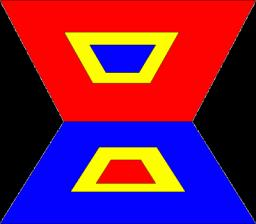Our examples were largely sent or created by pupils and students themselves. Therefore, we would be pleased if you could send us any errors you found, spelling mistakes, or rephasing the example. Thank you!

Leave us a comment of this math problem and its solution (i.e. if it is still somewhat unclear...):Be the first to comment!Tips to related online calculators

## Next similar math problems:

1. Trapezoid - RRFind the area of the right angled trapezoid ABCD with the right angle at the A vertex; a = 3 dm b = 5 dm c = 6 dm d = 4 dm
2. Isosceles trapezoid v3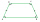In an isosceles trapezoid ABCD is the size of the angle β = 81° Determine size of angles α, γ and δ.
3. Isosceles trapezium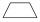Trapezoid YSED (YS||ED) is isosceles. The size of the angle at vertex Y is 17 degrees. Calculate the size of the angle at vertex E.
4. Triangle P2Can triangle have two right angles?
5. SimilarityAre two right triangles similar to each other if the first one has a acute angle 70° and second one has acute angle 20°?
6. Area of RT 2Calculate the area of right triangle whose legs have a length 5.8 cm and 5.8 cm.
7. Angles in triangle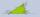Calculate the alpha angle in the triangle if beta is 61 degrees and 98 gamma degrees.
8. Two anglesThe triangles ABC and A'B'C 'are similar. In the ABC triangle, the two angles are 25° and 65°. Explain why in the triangle A'B'C 'is the sum of two angles of 90 degrees.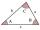The size of two internal angles of a triangle ABC are α=6/18π and β=7/18π. Calculate the size of the third angle.
10. 3-bracket 3Two angles in a triangle are 90° and 60°. Has triangle at least two equal sides?
11. Parallelogram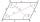In the parallelogram we know one internal angle 67°33`. Calculate the other internal angles.
12. Angles 1It is true neighboring angles have not common arm?
13. 3-bracketMay be the largest angle in the triangle less than 20°?
14. DiagonalCan a rhombus have the same length diagonal and side?
15. QuizQ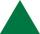An isosceles triangle has two sides of length 7 km and 39 km. How long is a third side?
16. MidpointsTriangle whose sides are midpoints of sides of triangle ABC has a perimeter 45. How long is perimeter of triangle ABC?
17. Obtuse angleWhich obtuse angle is creating clocks at 17:00?